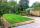# Square into three rectangles

Divide the square with a side length of 12 cm into three rectangles with have the same circumference so that these circumferences are as small as possible.

Result

a =  12
b =  3
c =  9
d =  6
o1 =  30
o2 =  30
o3 =  30
o4 =  32

#### Solution:

a=12
o1 = 2(a+b)
o2 = 2(c+d)
o3 = 2(c+d)
o1=o2
b+c=a
2d = 12
o4 = 2(a+a/3)

a = 12
2a+2b-o1 = 0
2c+2d-o2 = 0
2c+2d-o3 = 0
o1-o2 = 0
a-b-c = 0
2d = 12
8a-3o4 = 0

a = 12
b = 3
c = 9
d = 6
o1 = 30
o2 = 30
o3 = 30
o4 = 32

Calculated by our linear equations calculator.

Leave us a comment of this math problem and its solution (i.e. if it is still somewhat unclear...):

Showing 1 comment:Dr Math
so the solution is

1. 12*3 ...  a = 12 * b = 3
2. 9*6 ... c = 9 * d = 6
3. 9*6 ... c = 9 * d = 6

o1 is the circumference of the first, o2 the second, o3 the third rectangle
o4 is a circumference if the square is divided into same   12 * 4 rectangles#### Following knowledge from mathematics are needed to solve this word math problem:

Do you have a system of equations and looking for calculator system of linear equations?

## Next similar math problems:

1. GardensThe garden has the square shape with circumference 124 m. Divide it into two rectangular gardens, one should circumference 10 meters more than the second. What size will have a gardens?
2. PropertyThe length of the rectangle-shaped property is 8 meters less than three times of the width. If we increase the width 5% of a length and lendth reduce by 14% of the width it will increase the property perimeter by 13 meters. How much will the property cost
3. ParallelogramThe perimeter of the parallelogram is 417 cm. The length of one side is 1.7-times longer than the length of the shorter side. What is the length of sides of a parallelogram?
4. Rectangle SSPerimeter of a rectangle is 268 cm and its diagonal is 99.3 cm. Determine the dimensions of the rectangle.
5. Two squaresTwo squares whose sides are in the ratio 5:2 have sum of its perimeters 73 cm. Calculate the sum of area this two squares.
6. PlotThe length of the rectangle is 8 smaller than three times the width. If we increase the width by 5% of the length and the length is reduced by 14% of the width, the circumference of rectangle will be increased by 30 m. What are the dimensions of the rectan
7. RT leg and perimeterCalculate the length of the sides of a right triangle ABC with hypotenuse c when the length of a leg a= 84 and perimeter of the triangle o = 269.
8. Rectangle from stringString 12m. Make rectangle when one side is two times longer than its width.
9. RectangleThe width of the rectangle is 65% of its length. Perimeter of the rectangle is 132 cm. Determine the dimensions of the rectangle.
10. Rectangle - sides ratioCalculate area of rectangle whose sides are in ratio 3:13 and perimeter is 673.
11. Triangle - is RT?Triangle has a circumference of 90 cm. Side b is 1 cm longer than c, side c is 31 cm longer than side a. Calculate the length of sides and determine whether triangle is a right triangle.
12. Triangle ABCIn a triangle ABC side b measure 10 cm less than the side a and side b is half of the side c. Calculate the length of sides if the circumference of the triangle is 42 cm.
13. GardenThe garden has a rectangular shape and has a circumference of 130 m and area 800.25 m2. Calculate the dimensions of the garden.
14. Circle arcCircle segment has a circumference of 135.26 dm and 2096.58 dm2 area. Calculate the radius of the circle and size of central angle.
15. Isosceles triangleThe perimeter of an isosceles triangle is 112 cm. The length of the arm to the length of the base is at ratio 5:6. Find the triangle area.
16. ParallelogramParallelogram has sides lengths in the ratio 3: 4 and perimeter 2.8 meters. Determine the lengths of the sides.
17. Right triangle AlefThe obvod of a right triangle is 84 cm, the hypotenuse is 37 cm long. Determine the lengths of the legs.